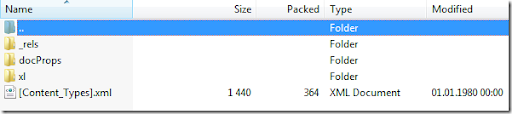## Thursday, November 4, 2010

### Reading Excel Sheets (xlsx) with .Net

The most common way to read Excel sheets up until recently was to use ADO.Net with the ACE OLEDB driver. It works, but you have to install the latest drivers etc., and isn’t it time to do it differently.
Third party solutions aside like Aspose, it’s possible to do this with all native .Net code. As many may or may not know, C# 3.0 introduced the System.IO.Packaging namespace used to work with Office Open Xml format, used by Office 2007 and newer. Files with docx, xlsx and pptx are all created in this format, and are basically zipped xml file structures. Rename a xlsx file to zip, and open it in your favorite zip browser and you will se something like this:Fortunately someone took the time to create an Excel project based on this new functionality. The project is called ExcelPackage, and can be found over at Codeplex. There are some samples there for reading and writing, but if you want to iterate over all cells in your workbook sheets, you have to play around a little bit. It’s not hard, but I put together this small sample to illustrate how you can output any cell with data in it from an Excel workbook.
The big trick was to select the dimension node from the sheet xml, since it’s not exposed as a property.
```public class Dimension
{
public int StartRow { get; set; }
public int StartColumn { get; set; }
public int EndRow { get; set; }
public int EndColumn { get; set; }
}

{
{
FileInfo excelFile = new FileInfo(@"C:\temp\book1.xlsx");
IterateExcelFile(excelFile, cell => Console.WriteLine(cell.CellAddress + ":" + cell.Value));
}

private void IterateExcelFile(FileInfo excelFile, Action<ExcelCell> operation)
{
using (ExcelPackage xlPackage = new ExcelPackage(excelFile))
{
foreach (ExcelWorksheet worksheet in xlPackage.Workbook.Worksheets)
{
var dimension = worksheet.Dimension();
var cells = from row in Enumerable.Range(dimension.StartRow, dimension.EndRow)
from column in Enumerable.Range(dimension.StartColumn, dimension.EndColumn)
where string.IsNullOrWhiteSpace(worksheet.Cell(row, column).Value) == false
select worksheet.Cell(row, column);

foreach (ExcelCell excelCell in cells)
{
operation(excelCell);
}
}
}
}
}

public static class ExcelHelper
{

public static Dimension Dimension(this ExcelWorksheet worksheet)
{
string range = worksheet.WorksheetXml.SelectSingleNode("//*[local-name()='dimension']").Attributes["ref"].Value;
string[] rangeCoordinates = range.Split(':');

var rowAndColumn = GetRowAndColumn(rangeCoordinates);
int startRow = rowAndColumn.Item1;
int startCol = rowAndColumn.Item2;

if (rangeCoordinates.Length == 1)
return new Dimension { StartRow = startRow, StartColumn = startCol, EndRow = startRow, EndColumn = startCol };

rowAndColumn = GetRowAndColumn(rangeCoordinates);
int endRow = rowAndColumn.Item1;
int endCol = rowAndColumn.Item2;
return new Dimension { StartRow = startRow, StartColumn = startCol, EndRow = endRow, EndColumn = endCol };
}

private static Tuple<int, int> GetRowAndColumn(string cellAddress)
{
int row = int.Parse(match.Groups["digit"].Value);
int col = ConvertFromExcelColumnName(match.Groups["letter"].Value);
return new Tuple<int, int>(row, col);
}

public static int ConvertFromExcelColumnName(string name)
{
name = name.ToUpper();
int result = 0;
for (int i = 0; i < name.Length - 1; i++)
{
int val = name[i] - 64;
int columnVal = (int)Math.Pow(26, name.Length - i - 1);
result += val * columnVal;
}
result += name[name.Length - 1] - 64;
return result;
}
}```